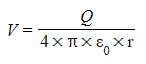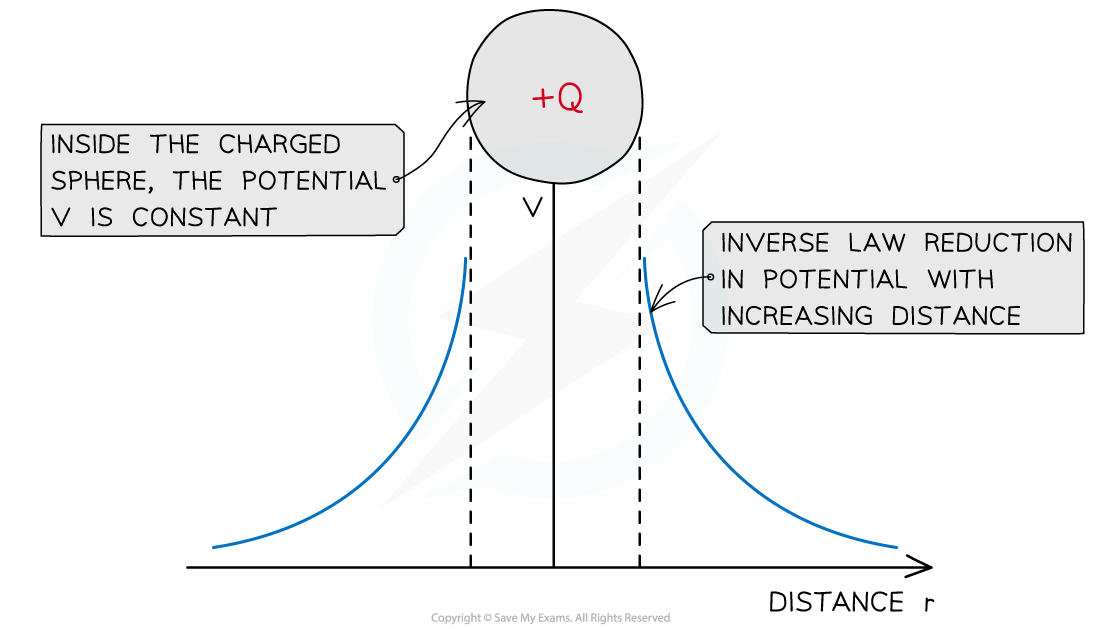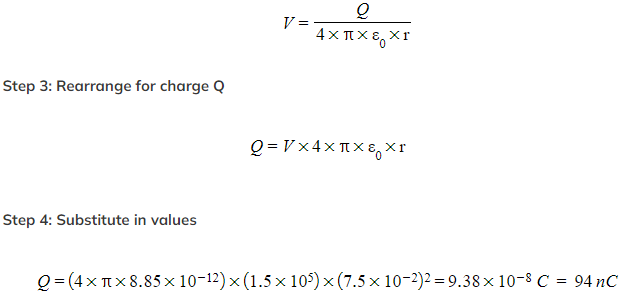# IB DP Physics: HL复习笔记10.2.4 Potential in a Charged Sphere

### Potential in a Charged Sphere

• The electric potential in the field due to a point charge is defined as:Where:
• V = the electric potential (V)
• Q = the point charge producing the potential (C)
• ε0 = permittivity of free space (F m-1)
• r = distance from the centre of the point charge (m)
• This equation shows that for a positive (+) charge:
• As the distance from the charge r decreases, the potential V increases
• This is because more work has to be done on a positive test charge to overcome the repulsive force
• For a negative (−) charge:
• As the distance from the charge r decreases, the potential V decreases
• This is because less work has to be done on a positive test charge thanks to the effect of the attractive force
• Unlike the gravitational potential equation, the minus sign in the electric potential equation will be included in the charge
• The electric potential changes according to an inverse square law with distanceThe potential changes as an inverse law with distance near a charged sphere

• Note: this equation still applies to a conducting sphere. The charge on the sphere is treated as if it concentrated at a point in the sphere from the point charge approximation

#### Worked Example

A Van de Graaf generator has a spherical dome of radius 15 cm. It is charged up to a potential of 240 kV. Calculate:

a) The charge stored on the dome

b) The potential at a distance of 30 cm from the dome

Part (a)

Step 1: Write down the known quantities

• Radius of the dome, r = 15 cm = 15 × 10-2 m
• Potential difference, V = 240 kV = 240 × 103 V

Step 2: Write down the equation for the electric potential due to a point chargePart (b)

Step 1: Write down the known quantities

• Q = charge stored in the dome = 4.0 μC = 4.0 × 10-6 C
• r = radius of the dome + distance from the dome = 15 + 30 = 45 cm = 45 × 10-2 m

Step 2: Write down the equation for electric potential due to a point chargeWorked Example

A metal sphere of diameter 15 cm is negatively charged. The electric field strength at the surface of the sphere is 1.5 × 105 V m-1. Determine the total surface charge of the sphere.

Step 1: Write down the known values

• Electric field strength, E = 1.5 × 105 V m-1
• Radius of sphere, r = 15 / 2 = 7.5 cm = 7.5 × 10-2 m
Step 2: Write out the equation for electric field strength